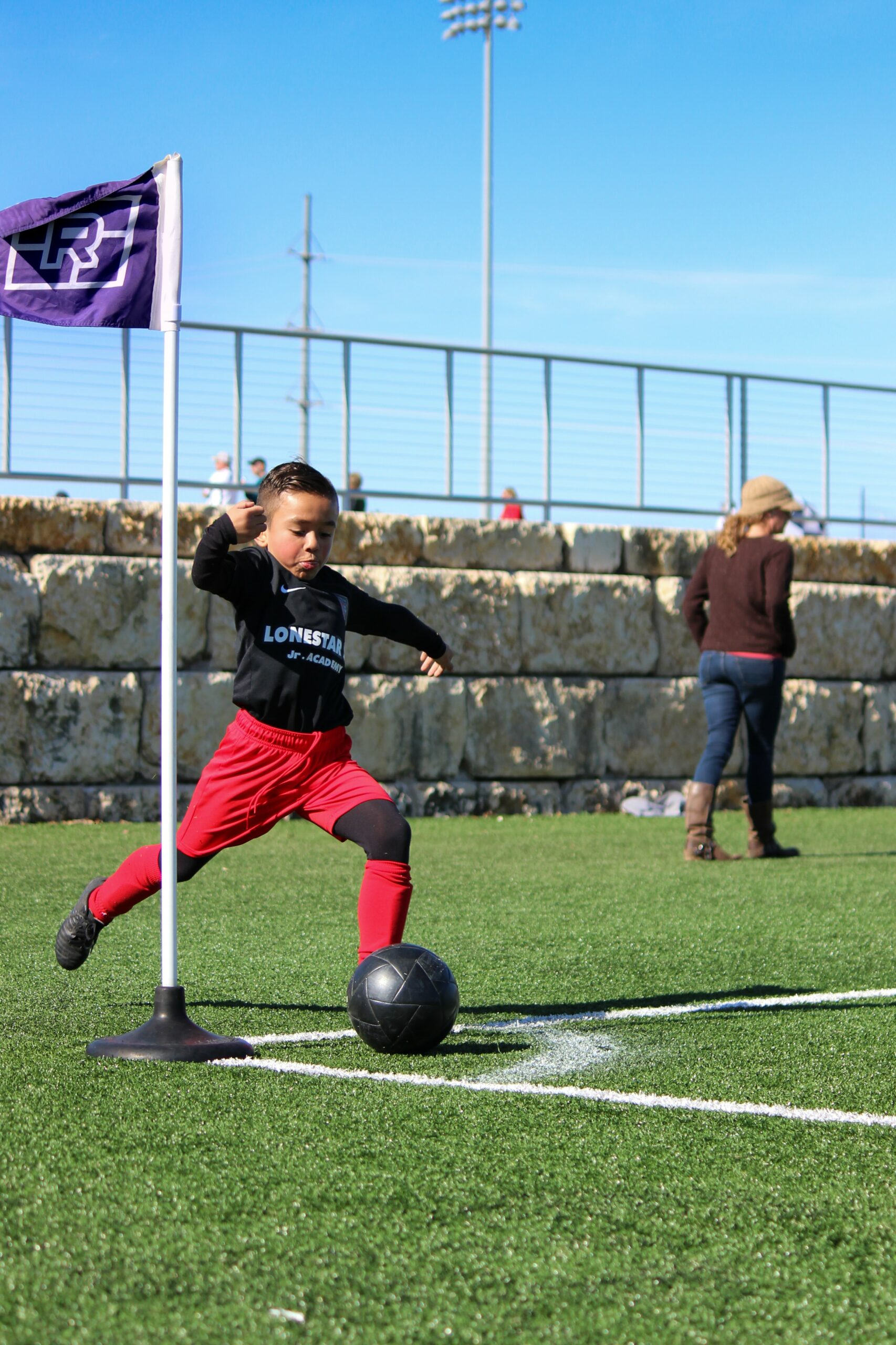It’s easy to forget to hydrate, especially when we are focused on protecting those around us due to Covid by wearing face masks.

So to support you in staying hydrated here is a simple way to establish a water goal to hit on a daily basis…

Fact: your body is made up of around 60% water so it goes without saying, keeping your body hydrated is VERY important.

Some of the benefits of drinking ENOUGH water are…

Improved organ function
Better circulation
Improved brain function/ focus
Increased nutrient absorption
Clear skin
Regular BMs💩
Increased energy🔥

So if you want to take advantage of all these benefits use the calculation below to find out the ideal amount of water you should be drinking DAILY👇

1. Take your weight (in pounds) and divide that by 2.2

2. Multiply that number depending on your age: If you’re younger than 30, multiply by 40. If you’re between 30-55, multiply by 35. If you’re older than 55, multiply by 30

3. Divide that sum by 28.3

Your total is how many ounces of water you should drink each day.

4. Divide that number by 8 to see your result in cups

Example: 140lb person/2.2= 63.63 × 35 = 2227

Then …

2227/28.3 = 78.7 oz/8 = 9.8 cups (round up to 10)

Now for every 45-60 minutes of exercise add 40oz onto the number above (this is a broad figure bc sweat rates vary), so…

78.7oz + 40oz = 118.7oz/8 = 14.83 cups (round to 15)

So if you think 8 cups per day was enough, think again!